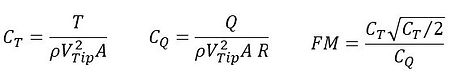top of page

# Suggested Data Processing

## Integrated Performance

Integrated  performance is a measure of the overall efficiency of a rotor system. Performance can be presented with plots of:

• CT versus collective pitch

• CQ versus collective pitch

• CQ versus CT

• Figure of Merit versus CT

where collective is measured at 75%R.

Detailed blade loading can be presented by section thrust and torque coefficients plotted as a function of r/R. These quantities are defined by:

Here c is the local chord.

## Pressure Distribution

Blade loading can be further detailed with plots of pressure coefficient versus x/c. Cp is defined by:

The following radial stations, (r/R) should be considered: 0.20, 0.40, 0.60, 0.70, 0.75, 0.80, 0.85, 0.90, 0.925, 0.95, 0.975, 0.99.

## Vortex Trajectory

Tip vortex trajectory plots should be provided as a function of wake age. The tip vortex trajectories should be plotted as z/ce from the blade tip trailing edge (zero displacement and zero wake age) versus wake age in degrees along with r/R versus wake age on a separate plot. The equivalent  thrust-weightedchord is defined as:

The equivalent chord is defined assuming the chord is constant from the cutout to zero radial position

## Vortex Core Size

The tip vortex core size should be plotted as core radius/ce versus wake age and the tip vortex circulation should be plotted as G/(ce WR) versus wake age.bottom of page